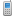MV Software Knowledge Bas… Modules»Inventory Control»Price Based on the Step Method

Tags:

# Price Based on the Step Method

Discount Table Master (Task 22 in the Inventory module) supports a pricing method for ‘cumulative’ or ‘step-pricing’.  A field on the table determines whether the discount is fixed or cumulative.  The field is labeled:  Fixed / Cume

Fixed: is the traditional discount method where the discount is determined by locating a fixed point in the table based on the quantity sold and applying the discount at that point to the entire quantity being sold.

Cume (for cumulative): sometimes referred to as:  STEP Pricing.  This process adds the dollar amounts for quantities at each break up to the point where the total sale quantity is reached. The total accumulated dollar value is then divided by the total quantity to get a ‘unit’ price.  This method is useful in situations where there is a setup (or other charges) that apply to the first unit of an item, but no subsequent ones. See below for a sample setup and the calculations:

01. Table name :           QG

02. Table descr:            CUME EXAMPLE

03. Break on D/Q:        Q

04. Amount type:          \$ (% L \$)

05. Fixed / Cume:         C

06. Quantity    :

07.      Price  :               75.00

08. Quantity    :             10

09.      Price  :               65.00

10. Quantity    :             20

11.      Price  :               50.00

Customer buys 10 of an item that has Quantity Discount Table QG on it (a G is on field 30 in Item Master) and will pay a total of \$740.00 (or \$74.00 each)  Based on the table QG, the calculation is determined by:

The first 9 of the item:               9 @ \$75.00     =          \$ 675.00

The 10th  item:                          1 @ \$65.00    =               65.00

Totals:                                      10                                \$ 740 .00        or   \$74.00 each

*The 2nd Quantity Break is 10, therefore first 9 are priced at \$75 each, then the 10th item falls into the ‘next’ Quantity Break @ \$65.00 each.

Another example:  a customer buys 25 of the same item, the price calculation of a \$65.00 selling price for each is determined as follows:

The first 9 of the item:                 9 @ \$75.00   =          \$ 675.00

10 thru 19 of the item:               10 @ \$65.00   =          \$ 650.00

20 thru 25 of the item:                 @ \$50.00   =          \$ 300.00

Totals:                                      25                               \$1625.00         or  \$65.00 each